Next: Waveguides Up: Dispersive Waves Previous: Faraday Rotation

# Electromagnetic Wave Propagation in Conductors

A so-called Ohmic conductor is a medium that satisfies Ohm's law, which can be written in the form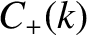(862)

where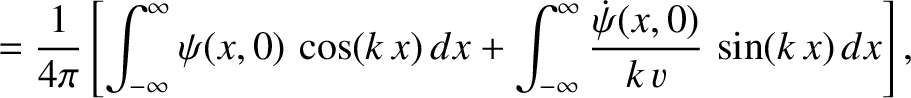is the current density (i.e., the current per unit area),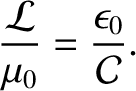the electric field-strength, and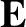a constant known as the electrical conductivity of the medium in question. The-directed propagation of a plane electromagnetic wave, linearly polarized in the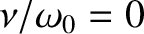-direction, through an Ohmic conductor of conductivityis governed by (see Appendix C)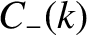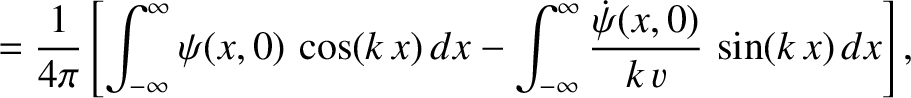(863)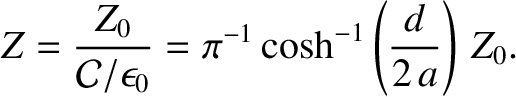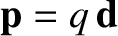(864)

For a so-called good conductor, which satisfies the inequality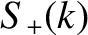, the first term on the left-hand side of Equation (863) is negligible with respect to the second term, and the previous two equations reduce to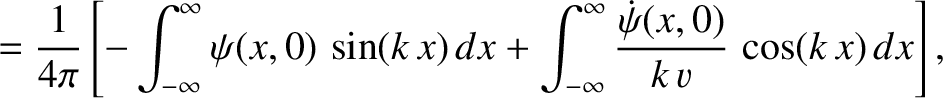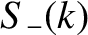(865)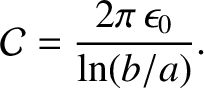(866)

These equations can be solved to give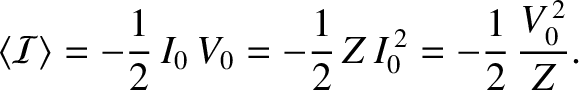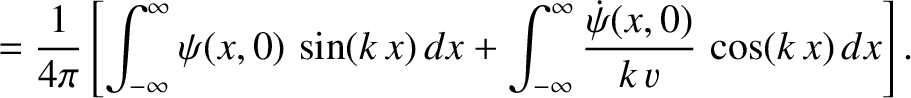(867)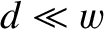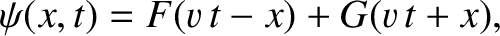(868)

where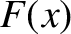(869)

and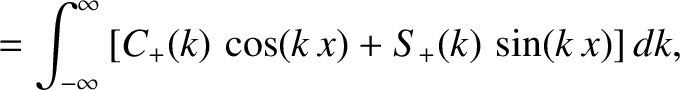(870)

(See Exercise 7.) Equations (867) and (868) indicate that the amplitude of an electromagnetic wave propagating through a conductor decays exponentially on a characteristic lengthscale,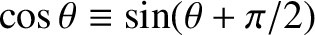, that is known as the skin-depth. Consequently, an electromagnetic wave cannot penetrate more than a few skin-depths into a conducting medium. The skin-depth is smaller at higher frequencies. This implies that high frequency waves penetrate a shorter distance into a conductor than low frequency waves.

Consider a typical metallic conductor such as Copper, whose electrical conductivity at room temperature is about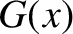(Wikipedia contributors 2012). Copper, therefore, acts as a good conductor for all electromagnetic waves of frequency below about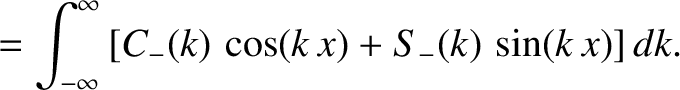. The skin-depth in Copper for such waves is thus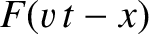(871)

It follows that the skin-depth is about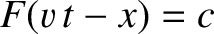at 1Hz, but only about 2mm at 1kHz. This gives rise to the so-called skin effect in copper wires, by which an oscillating electromagnetic signal of increasing frequency, transmitted along such a wire, is confined to an increasingly narrow layer (whose thickness is of order the skin-depth) on the surface of the wire.

The conductivity of sea-water is only about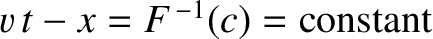(Wikipedia contributors 2012). However, this is still sufficiently high for sea-water to act as a good conductor for all radio frequency electromagnetic waves (i.e.,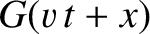GHz). The skin-depth at 1MHz (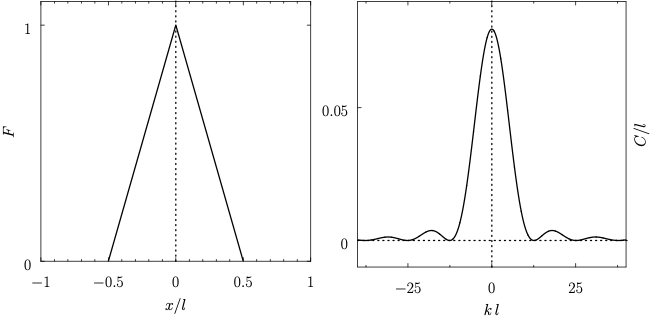m) is about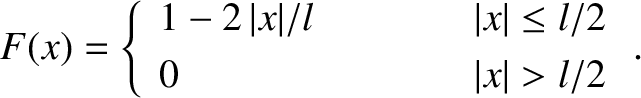m, whereas that at 1kHz (km) is still only about 7m. This obviously poses quite severe restrictions for radio communication with submerged submarines. Either the submarines have to come quite close to the surface to communicate (which is dangerous), or the communication must be performed with extremely low frequency (ELF) waves (i.e.,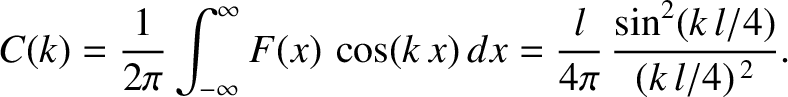Hz). Unfortunately, such waves have very large wavelengths (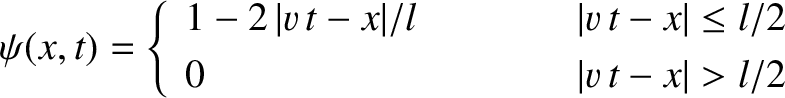), which means that they can only be efficiently generated by gigantic antennas.

According to Equation (868), the phase of the magnetic component of an electromagnetic wave propagating through a good conductor lags that of the electric component by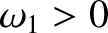radians. It follows that the mean energy flux into the conductor takes the form (see Appendix C)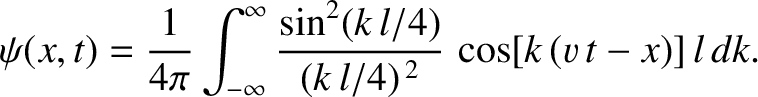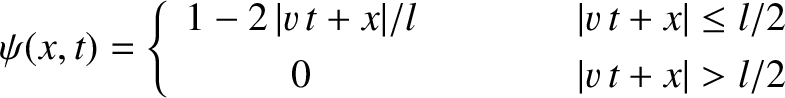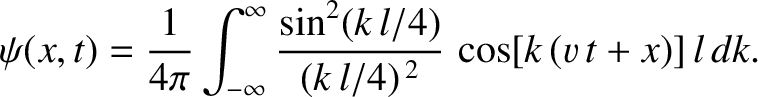(872)

where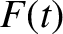is the amplitude of the electric component of the wave. The fact that the mean energy flux is positive indicates that some of the wave energy is absorbed by the conductor. In fact, the absorbed energy corresponds to the energy lost due to Joule heating in the conductor. (See Exercise 10.)

According to Equation (870), the impedance of a good conductor is far less than that of a vacuum (i.e.,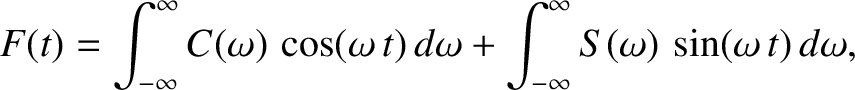). This implies that the ratio of the magnetic to the electric components of an electromagnetic wave propagating through a good conductor is far larger than that of a wave propagating through a vacuum.

Suppose that the region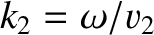is a vacuum, and the region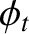is occupied by a good conductor of conductivity. Consider a linearly polarized plane wave normally incident on the interface. Let the wave electric and magnetic fields in the vacuum region take the form of the incident and reflected waves specified in Equations (812) and (813). The wave electric and magnetic fields in the conductor are written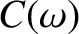(873)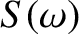(874)

where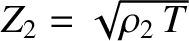is the amplitude of the evanescent wave that penetrates into the conductor,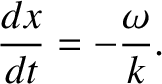is the phase of this wave with respect to the incident wave, and(875)

The appropriate matching conditions are the continuity of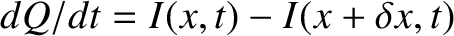and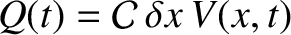at the vacuum/conductor interface (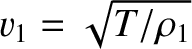). (See Appendix C.) In other words,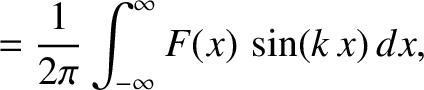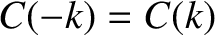(876)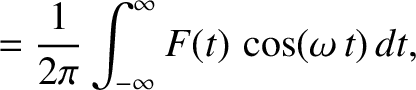(877)

Equations (876) and (877), which must be satisfied at all times, can be solved, in the limit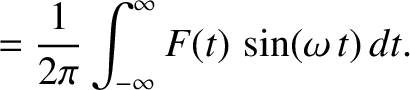, to give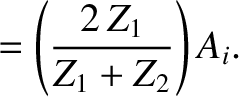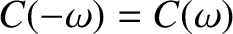(878)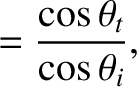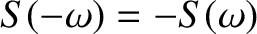(879)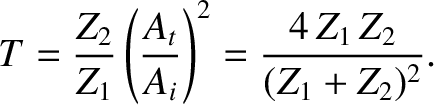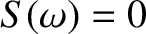(880)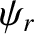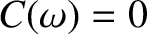(881)

(See Exercise 8.) Hence, the coefficient of reflection becomes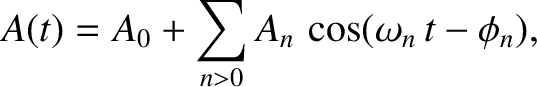(882)

According to the previous analysis, a good conductor reflects a normally incident electromagnetic wave with a phase shift of almost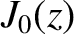radians (i.e.,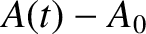). The coefficient of reflection is just less than unity, indicating that, while most of the incident energy is reflected by the conductor, a small fraction of it is absorbed.

High quality metallic mirrors are generally coated in Silver, whose conductivity is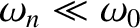(Wikipedia contributors 2012). It follows, from Equation (882), that at optical frequencies (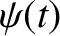) the coefficient of reflection of a silvered mirror is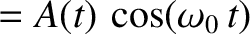. This implies that about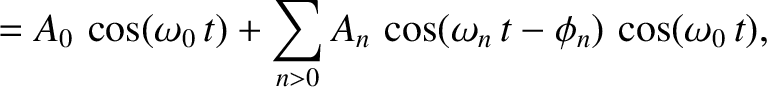of the light incident on the mirror is absorbed, rather than being reflected. This rather severe light loss can be problematic in instruments, such as astronomical telescopes, that are used to view faint objects.Next: Waveguides Up: Dispersive Waves Previous: Faraday Rotation
Richard Fitzpatrick 2013-04-08